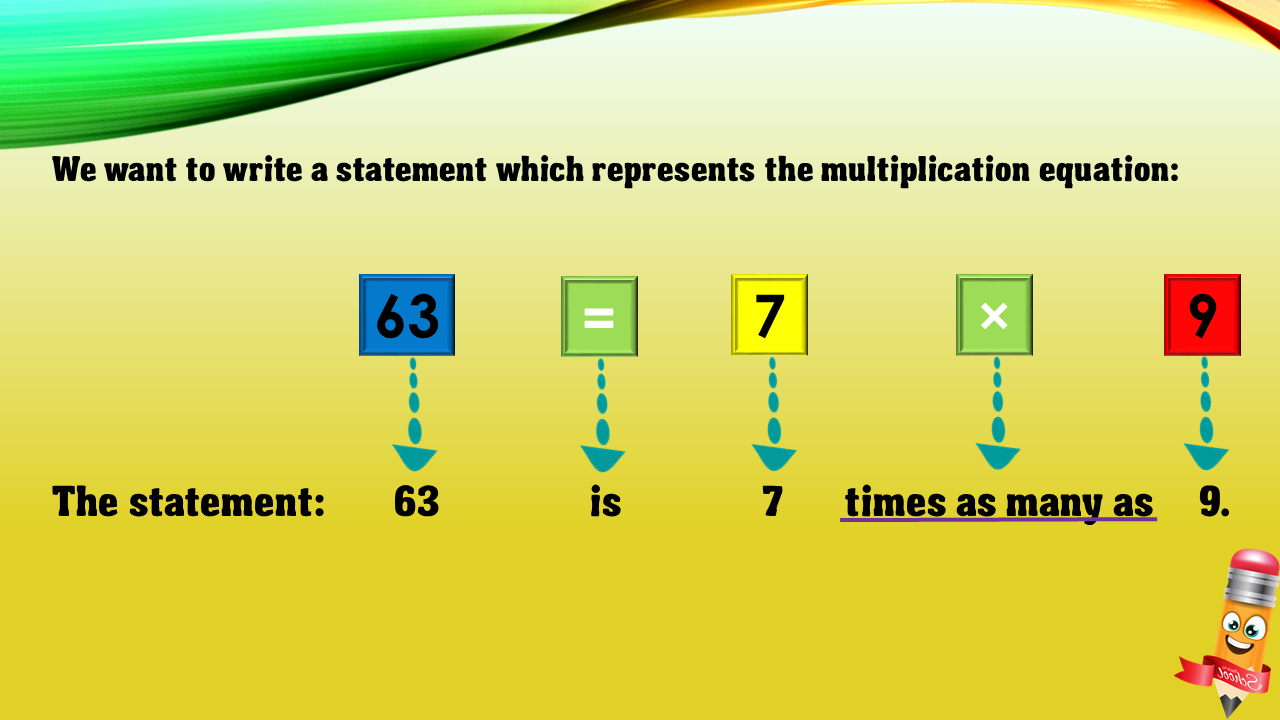1
visibility

Choose one statement which represents the equation: 63 = 7×9 ?

• A

63 is 7 times as many as 9.

• B

63 is 7 more than 9.

• C

7 is 9 less than 63.

• D

63 times as many as 7 is 9.